Examples

Chapter 4 Class 11 Mathematical Induction
Serial order wise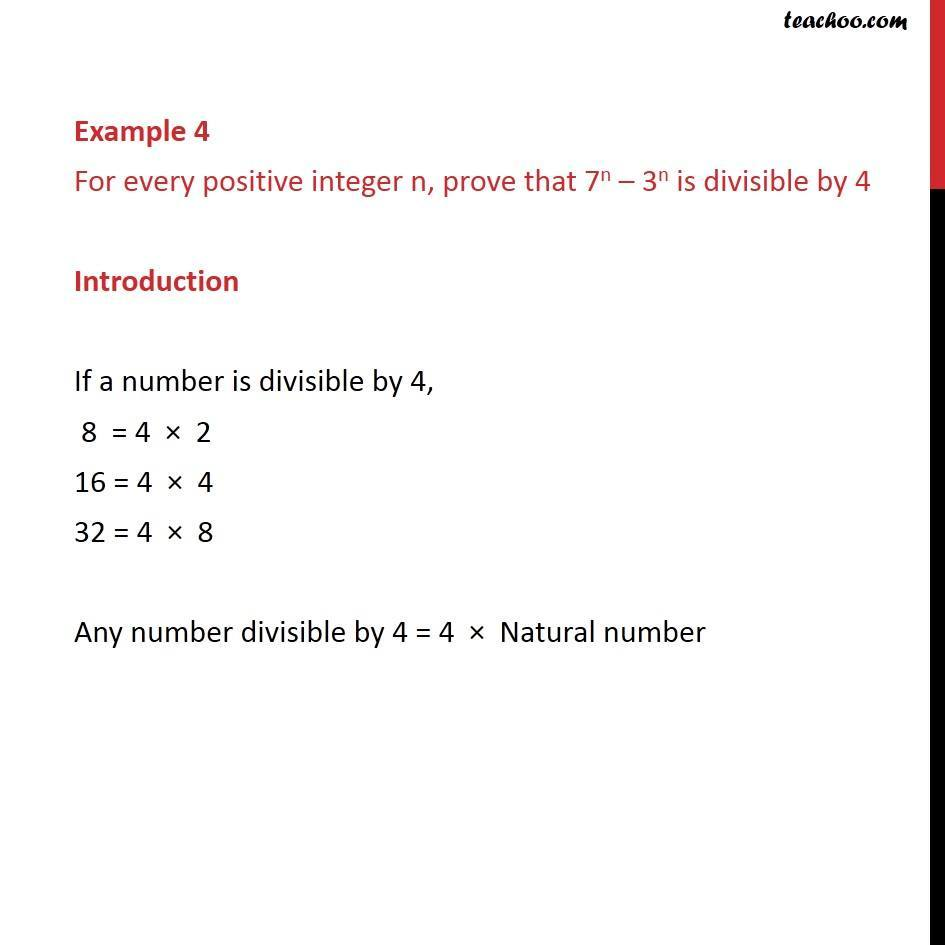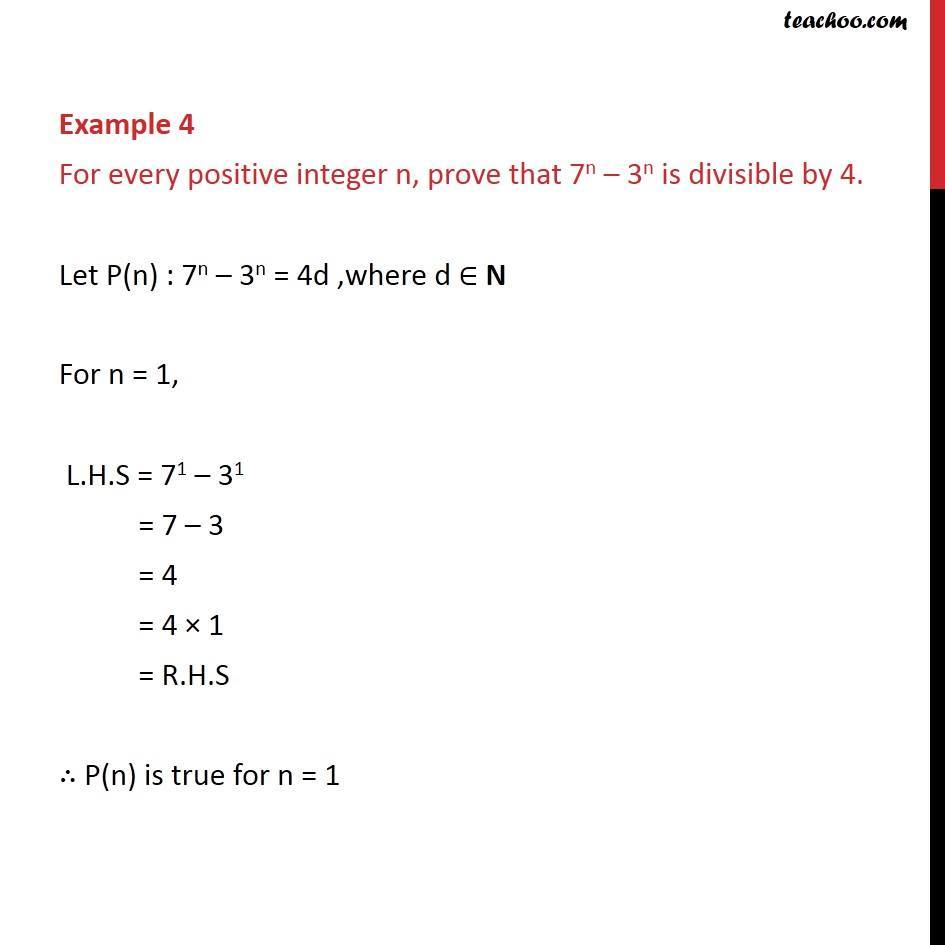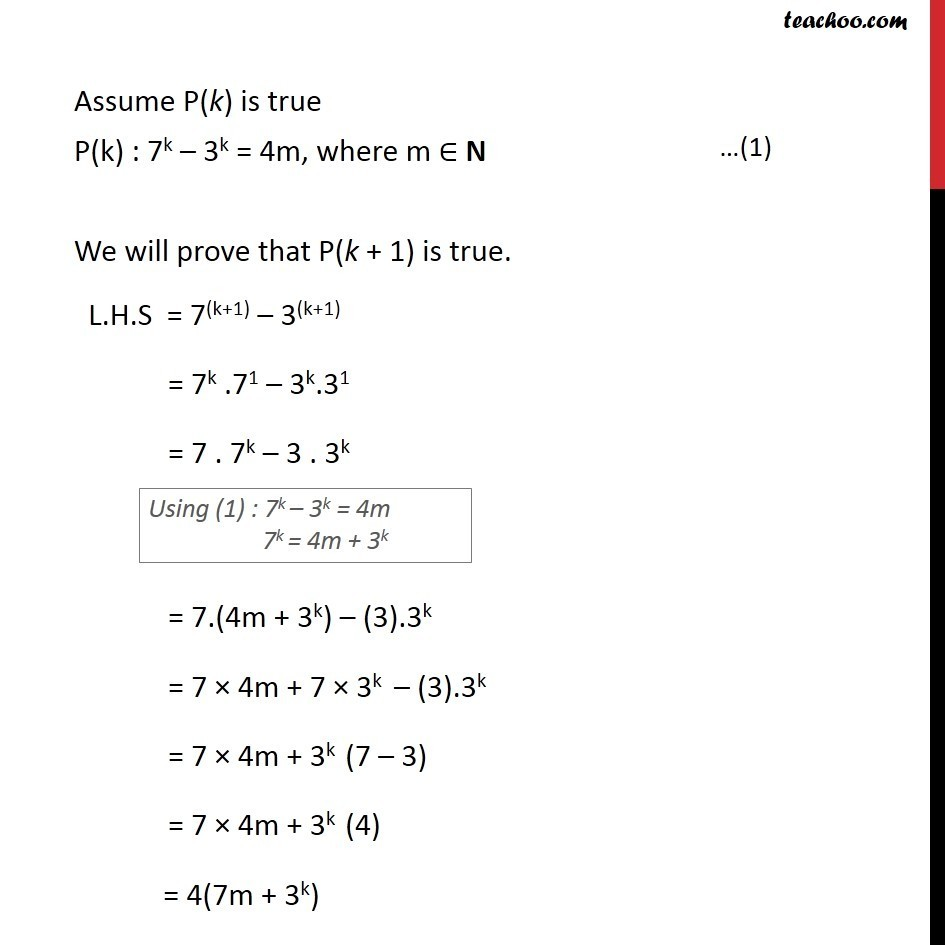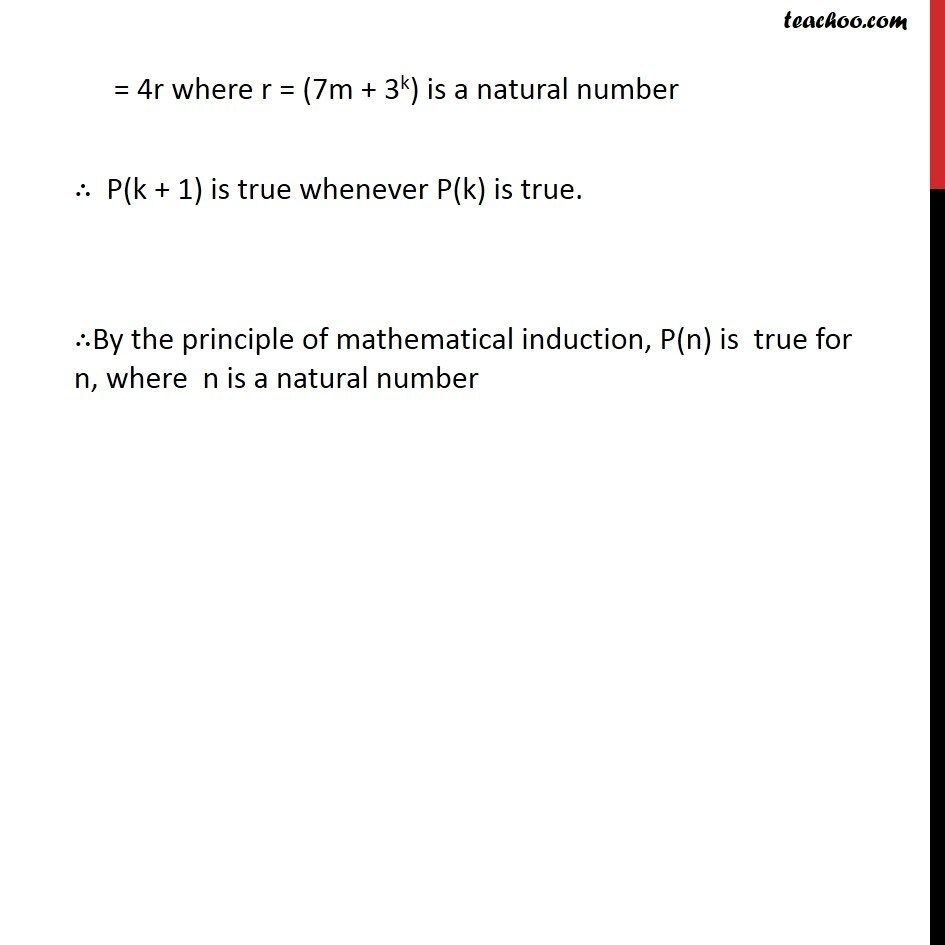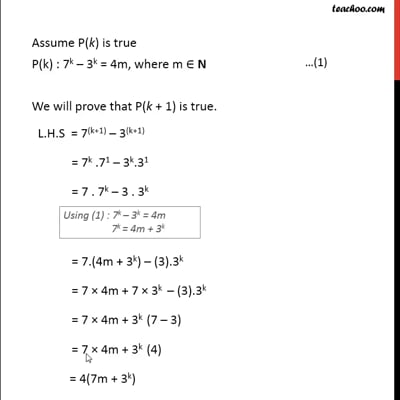This video is only available for Teachoo black users

Introducing your new favourite teacher - Teachoo Black, at only ₹83 per month

### Transcript

Example 4 For every positive integer n, prove that 7n – 3n is divisible by 4 Introduction If a number is divisible by 4, 8 = 4 × 2 16 = 4 × 4 32 = 4 × 8 Any number divisible by 4 = 4 × Natural number Example 4 For every positive integer n, prove that 7n – 3n is divisible by 4. Let P(n) : 7n – 3n = 4d ,where d ∈ N For n = 1, L.H.S = 71 – 31 = 7 – 3 = 4 = 4 × 1 = R.H.S ∴ P(n) is true for n = 1 Assume P(k) is true P(k) : 7k – 3k = 4m, where m ∈ N We will prove that P(k + 1) is true. L.H.S = 7(k+1) – 3(k+1) = 7k .71 – 3k.31 = 7 . 7k – 3 . 3k = 7.(4m + 3k) – (3).3k = 7 × 4m + 7 × 3k – (3).3k = 7 × 4m + 3k (7 – 3) = 7 × 4m + 3k (4) = 4(7m + 3k) = 4r where r = (7m + 3k) is a natural number ∴ P(k + 1) is true whenever P(k) is true. ∴By the principle of mathematical induction, P(n) is true for n, where n is a natural number